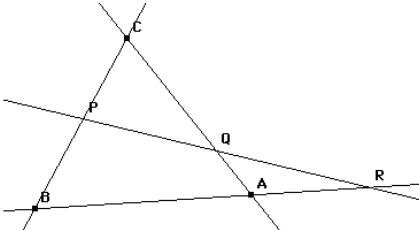# Menelaus's theoremIf a line meets the sides $BC, CA$ and $AB$ of a triangle in points $P, Q$ and $R$ then the product of the ratios

$\Large \frac {BP}{PC}.\frac {CQ}{QA}.\frac {AR}{RB}$ is -1

Conversely, if points $P, Q$ and $R$ are on the three sides of the triangle and this ratio is -1 then the three points are collinear.